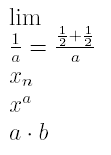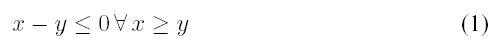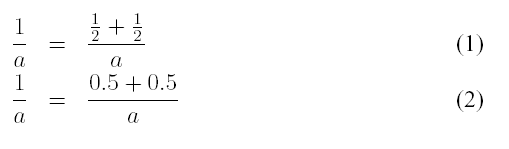Back to examples   Back to overview   Forward to error documentation 1

## Short documentation for course 1

• LaTeX example
• Special character
• Writing command
• Lists
• unnummbered lists
• numbered lists
• Tables
• Mathematics
• produce a ps file
• produce a pdf file

• ### Example of a LaTeX document

A small Latex document

\documentclass[12pt,twoside,a4paper]{article}
\begin{document}
a small \LaTeX \ document
\end{document}

This code spends the following:
A small LaTeX document

### Special indications

 \ Escape character: masks special character and introduces commands { } if arguments enclose, text blocks form etc. % Comment symbol: The remainder of the line is ignored $enclose mathematical formula in pairs inside of text ^ _ exponent and index in mathe & depending upon context - tabulator ~ Protected blank. ### Overview of the commands \textbf{Bold} Bold \textit{italic} italic \textrm{Text} rm = roman \emph{emphasized} emphasized ### Lists #### unnumbered lists  \begin{itemize} \item one point of pass \item still another one point of pass \end{itemize}  • One point of pass • Still another one point of pass #### numbered lists  \begin{enumerate} \item one point of pass \item still another one point of pass \end{enumerate}  1. One point of pass 2. Still another one point of pass ### Tables  \begin{tabular}{|l|c|r|p{1.5 cm }|} \hline left & centers & right & width \\ l & C & r & p \\ \hline \end{tabular}   left centered right Width l C r p  l left justified r right-justified C centered p firm width with line-makeup | vertical line \hline horizontal line ### Mathematics Mathematics packages:  \usepackage{amssymb,amsmath} $\lim \frac{1}{a } = \frac{\frac{1}{2 } + \frac{1}{2}}{a}$\\$x_{n}$\\$x^{a}$\\$a \cdot b \$ \\\begin{displaymath}
XY \leq 0 \, \forall \, x \geq y
\end{displaymath}\begin{equation}
XY \leq 0 \, \forall \, x \geq y
\end{equation}\begin{eqnarray}
\frac{1}{a} & = & \frac{\frac{1}{2} + \frac{1}{2}}{a} \\
\frac{1}{a} & = & \frac{0.5 + 0.5}{a}
\end{eqnarray}### ps file with LaTeX

1. compile with latex test.tex
2. look at with xdvi test.dvi
3. convert with dvips test.dvi into ps file
4. look at with gv test.ps

### pdf file with LaTeX

1. compile with pdflatex test.tex
2. look at with xpdf test pdf
Back to examples   Back to overview   Forward to error documentation 1
Sascha Frank
Last modified: Wed Aug 31 20:37:53 MEST 2005